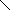# MINLPLib

### A Library of Mixed-Integer and Continuous Nonlinear Programming Instances

#### Instance: ball_mk2_10

```A simple MINLP with a feasible set described by a ball.
The basic model over which these variations are made is:
min  sum_i=1^n x_i
s.t. sum_i=1^n (x_i - 0.5)^2 <= (n-1)/4
x integer between -1 and 1.
Obvisouly, this problem is infeasible and has no solution.
It can be shown that any outer-approximation based method will need 2^n linear inequalities to show infeasibility, see reference.
In this instance, the ball is slightly moved to contain the point 0.```
 Formatsⓘ ams gms lp mod nl osil pip Primal Boundsⓘ 0.00000000 p1 ( gdx sol ) (infeas: 0) Dual Boundsⓘ 0.00000000 (ALPHAECP)-0.00000000 (ANTIGONE)0.00000000 (BARON)0.00000000 (BONMIN)0.00000000 (COUENNE)0.00000000 (LINDO)0.00000000 (SCIP) Referencesⓘ Hijazi, Hassan, Bonami, Pierre, and Ouorou, Adam, An Outer-Inner Approximation for Separable Mixed-Integer Nonlinear Programs, INFORMS Journal on Computing, 26:1, 2014, 31-44. Sourceⓘ Pierre Bonami Applicationⓘ Geometry Added to libraryⓘ 11 Sep 2017 Problem typeⓘ IQCP #Variablesⓘ 10 #Binary Variablesⓘ 0 #Integer Variablesⓘ 10 #Nonlinear Variablesⓘ 10 #Nonlinear Binary Variablesⓘ 0 #Nonlinear Integer Variablesⓘ 10 Objective Senseⓘ min Objective typeⓘ linear Objective curvatureⓘ linear #Nonzeros in Objectiveⓘ 10 #Nonlinear Nonzeros in Objectiveⓘ 0 #Constraintsⓘ 1 #Linear Constraintsⓘ 0 #Quadratic Constraintsⓘ 1 #Polynomial Constraintsⓘ 0 #Signomial Constraintsⓘ 0 #General Nonlinear Constraintsⓘ 0 Operands in Gen. Nonlin. Functionsⓘ Constraints curvatureⓘ convex #Nonzeros in Jacobianⓘ 10 #Nonlinear Nonzeros in Jacobianⓘ 10 #Nonzeros in (Upper-Left) Hessian of Lagrangianⓘ 10 #Nonzeros in Diagonal of Hessian of Lagrangianⓘ 10 #Blocks in Hessian of Lagrangianⓘ 10 Minimal blocksize in Hessian of Lagrangianⓘ 1 Maximal blocksize in Hessian of Lagrangianⓘ 1 Average blocksize in Hessian of Lagrangianⓘ 1.0 #Semicontinuitiesⓘ 0 #Nonlinear Semicontinuitiesⓘ 0 #SOS type 1ⓘ 0 #SOS type 2ⓘ 0 Infeasibility of initial pointⓘ 0 Sparsity JacobianⓘSparsity Hessian of Lagrangianⓘ```\$offlisting
*
*  Equation counts
*      Total        E        G        L        N        X        C        B
*          2        1        0        1        0        0        0        0
*
*  Variable counts
*                   x        b        i      s1s      s2s       sc       si
*      Total     cont   binary  integer     sos1     sos2    scont     sint
*         11        1        0       10        0        0        0        0
*  FX      0
*
*  Nonzero counts
*      Total    const       NL      DLL
*         21       11       10        0
*
*  Solve m using MINLP minimizing objvar;

Variables  objvar,i2,i3,i4,i5,i6,i7,i8,i9,i10,i11;

Integer Variables  i2,i3,i4,i5,i6,i7,i8,i9,i10,i11;

Equations  e1,e2;

e1..    objvar + i2 + i3 + i4 + i5 + i6 + i7 + i8 + i9 + i10 + i11 =E= 0;

e2.. sqr(i10) - 0.987420882906575*i10 + sqr(i9) - 0.987420882906575*i9 + sqr(i8
) - 0.987420882906575*i8 + sqr(i7) - 0.987420882906575*i7 + sqr(i6) -
0.987420882906575*i6 + sqr(i5) - 0.987420882906575*i5 + sqr(i4) -
0.987420882906575*i4 + sqr(i3) - 0.987420882906575*i3 + sqr(i2) -
0.987420882906575*i2 + sqr(i11) - 0.987420882906575*i11 =L= 0;

* set non-default bounds
i2.lo = -1; i2.up = 1;
i3.lo = -1; i3.up = 1;
i4.lo = -1; i4.up = 1;
i5.lo = -1; i5.up = 1;
i6.lo = -1; i6.up = 1;
i7.lo = -1; i7.up = 1;
i8.lo = -1; i8.up = 1;
i9.lo = -1; i9.up = 1;
i10.lo = -1; i10.up = 1;
i11.lo = -1; i11.up = 1;

Model m / all /;

m.limrow=0; m.limcol=0;
m.tolproj=0.0;

\$if NOT '%gams.u1%' == '' \$include '%gams.u1%'

\$if not set MINLP \$set MINLP MINLP
Solve m using %MINLP% minimizing objvar;

```

Last updated: 2019-02-14 Git hash: a71254dc